# 演练：创建和使用自己的动态链接库 (C++)

• 在 Visual Studio 中创建 DLL 项目。

• 将导出的函数和变量添加到该 DLL。

• 在 Visual Studio 中创建一个控制台应用项目。

• 在该控制台应用中使用从 DLL 导入的函数和变量。

• 运行已完成的应用。

## 先决条件

• 运行 Microsoft Windows 7 或更高版本的计算机。 建议使用最新版本的 Windows 获得最佳开发体验。
• Visual Studio 的副本。 有关如何下载和安装 Visual Studio 的信息，请参阅安装 Visual Studio。 运行安装程序时，请务必选中“使用 C++ 的桌面开发” 工作负载。 如果在安装 Visual Studio 时未安装此工作负载，请不要担心。 可以再次运行安装程序并立即安装。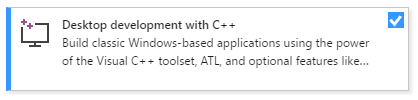• Visual Studio 的副本。 有关如何下载和安装 Visual Studio 2015 的信息，请参阅安装 Visual Studio 2015。 由于默认不安装 C++ 编译器和工具，请使用“自定义”安装进行安装 。
• 了解使用 Visual Studio IDE 的基础知识。 如果你之前使用过 Windows 桌面应用，可能具备一定的相关知识。 有关简介，请参阅 Visual Studio IDE 功能导览

• 了解足够的 C++ 语言基础知识以供继续操作。 别担心，我们不会执行过于复杂的操作。

## 创建 DLL 项目

### 在 Visual Studio 2019 中创建 DLL 项目

1. 在菜单栏上，选择“文件”>“新建”>“项目”，打开“创建新项目”对话框 。2. 在对话框顶部，将“语言”设置为“C++”，将“平台”设置为“Windows”，并将“项目类型”设置为“库”。

3. 从经过筛选的项目类型列表中，选择“动态链接库(DLL)”，然后选择“下一步” 。

4. 在“配置新项目”页面，在“项目名称”框中输入“MathLibrary”，以指定项目的名称 。 保留默认“位置”和“解决方案名称”值 。 将“解决方案”设置为“创建新解决方案” 。 如果“将解决方案和项目放在同一目录中”已选中，则取消选中 。

5. 选择“创建” 按钮创建项目。### 在 Visual Studio 2017 中创建 DLL 项目

1. 在菜单栏上，选择“文件”>“新建”>“项目”，打开“新建项目”对话框

2. 在“新建项目”对话框的左窗格中，选择“已安装”>“Visual C++”>“Windows 桌面” 。 在中心窗格中，选择“动态链接库(DLL)” 。 在“名称”框中输入“MathLibrary”，以指定项目的名称 。 保留默认“位置”和“解决方案名称”值 。 将“解决方案”设置为“创建新解决方案” 。 选中“创建解决方案的目录”（如果未选中） 。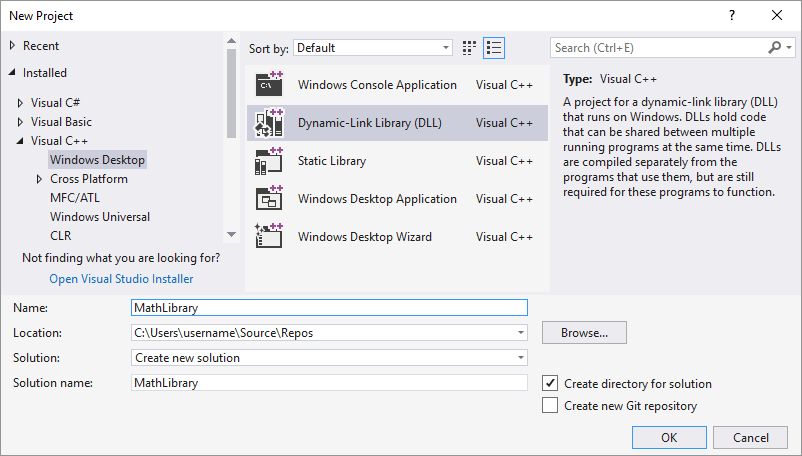3. 选择 “确定” 按钮，创建单元测试项目。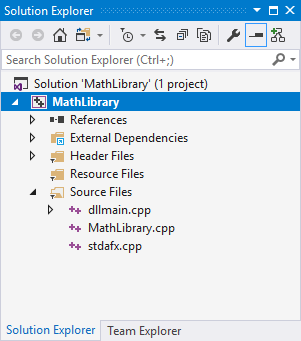### 在 Visual Studio 2015 及更早版本中创建 DLL 项目

1. 在菜单栏上，依次选择“文件”>“新建”>“项目”。

2. 在“新建项目” 对话框的左窗格中，展开“已安装” >“模板” ，并选择“Visual C++” ，然后在中心窗格中，选择“Win32 控制台应用程序” 。 在“名称”编辑框中输入“MathLibrary”，以指定项目的名称 。 保留默认“位置”和“解决方案名称”值 。 将“解决方案”设置为“创建新解决方案” 。 选中“创建解决方案的目录”（如果未选中） 。3. 选择“确定” 按钮以关闭“新建项目” 对话框并启动“Win32 应用程序向导” 。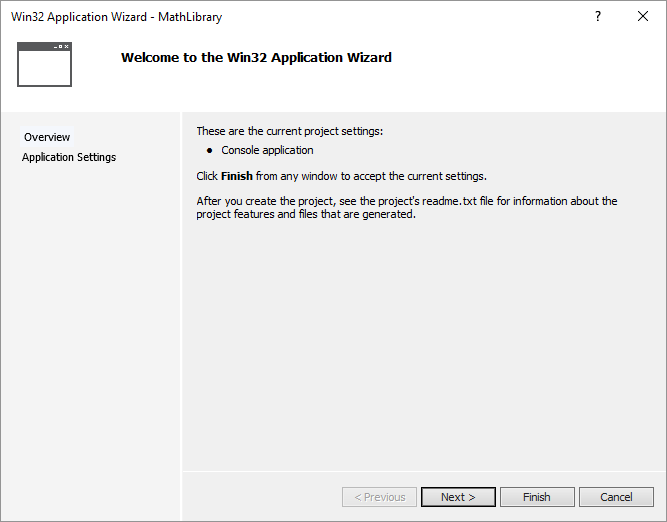4. 选择“下一步”按钮 。 在“应用程序设置”页的“应用程序类型”下，选择“DLL” 。5. 选择 “完成” 按钮创建项目。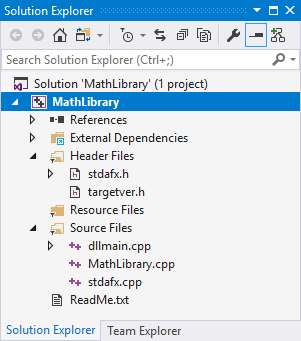### 将头文件添加到 DLL

1. 若要为函数创建头文件，请在菜单栏上选择“项目”>“添加新项” 。

2. 在“添加新项”对话框的左窗格中，选择“Visual C++” 。 在中间窗格中，选择 “头文件(.h)” 。 指定 MathLibrary.h 作为头文件的名称 。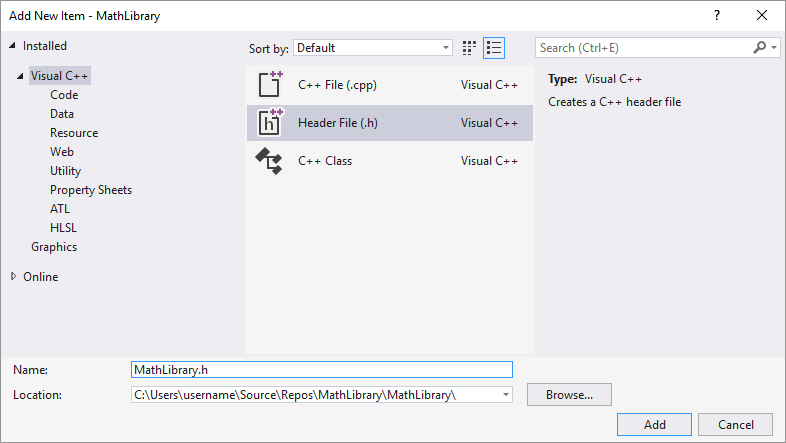3. 选择“添加” 按钮以生成一个空白头文件，该文件显示在新的编辑器窗口中。4. 将头文件的内容替换为以下代码：

``````// MathLibrary.h - Contains declarations of math functions
#pragma once

#ifdef MATHLIBRARY_EXPORTS
#define MATHLIBRARY_API __declspec(dllexport)
#else
#define MATHLIBRARY_API __declspec(dllimport)
#endif

// The Fibonacci recurrence relation describes a sequence F
// where F(n) is { n = 0, a
//               { n = 1, b
//               { n > 1, F(n-2) + F(n-1)
// for some initial integral values a and b.
// If the sequence is initialized F(0) = 1, F(1) = 1,
// then this relation produces the well-known Fibonacci
// sequence: 1, 1, 2, 3, 5, 8, 13, 21, 34, ...

// Initialize a Fibonacci relation sequence
// such that F(0) = a, F(1) = b.
// This function must be called before any other function.
extern "C" MATHLIBRARY_API void fibonacci_init(
const unsigned long long a, const unsigned long long b);

// Produce the next value in the sequence.
// Returns true on success and updates current value and index;
// false on overflow, leaves current value and index unchanged.
extern "C" MATHLIBRARY_API bool fibonacci_next();

// Get the current value in the sequence.
extern "C" MATHLIBRARY_API unsigned long long fibonacci_current();

// Get the position of the current value in the sequence.
extern "C" MATHLIBRARY_API unsigned fibonacci_index();
``````

### 向 DLL 添加实现

1. 在“解决方案资源管理器”中，右键单击“源文件”节点并选择“添加”>“新建项目” 。 使用上一步中添加新头文件的相同方式，创建名为 MathLibrary.cpp 的新 .cpp 文件 。

2. 在编辑器窗口中，选择 MathLibrary.cpp 的选项卡（如果已打开）。 如果未打开，请在“解决方案资源管理器”中，双击 MathLibrary 项目的“Source Files”文件夹中的 MathLibrary.cpp，将其打开 。

3. 在编辑器中，将 MathLibrary.cpp 文件的内容替换为以下代码：

``````// MathLibrary.cpp : Defines the exported functions for the DLL.
#include "pch.h" // use stdafx.h in Visual Studio 2017 and earlier
#include <utility>
#include <limits.h>
#include "MathLibrary.h"

// DLL internal state variables:
static unsigned long long previous_;  // Previous value, if any
static unsigned long long current_;   // Current sequence value
static unsigned index_;               // Current seq. position

// Initialize a Fibonacci relation sequence
// such that F(0) = a, F(1) = b.
// This function must be called before any other function.
void fibonacci_init(
const unsigned long long a,
const unsigned long long b)
{
index_ = 0;
current_ = a;
previous_ = b; // see special case when initialized
}

// Produce the next value in the sequence.
// Returns true on success, false on overflow.
bool fibonacci_next()
{
// check to see if we'd overflow result or position
if ((ULLONG_MAX - previous_ < current_) ||
(UINT_MAX == index_))
{
return false;
}

// Special case when index == 0, just return b value
if (index_ > 0)
{
// otherwise, calculate next sequence value
previous_ += current_;
}
std::swap(current_, previous_);
++index_;
return true;
}

// Get the current value in the sequence.
unsigned long long fibonacci_current()
{
return current_;
}

// Get the current index position in the sequence.
unsigned fibonacci_index()
{
return index_;
}
``````
1. 在编辑器窗口中，选择 MathLibrary.cpp 的选项卡（如果已打开）。 如果未打开，请在“解决方案资源管理器”中，双击 MathLibrary 项目的“Source Files”文件夹中的 MathLibrary.cpp，将其打开 。

2. 在编辑器中，将 MathLibrary.cpp 文件的内容替换为以下代码：

``````// MathLibrary.cpp : Defines the exported functions for the DLL.
#include "stdafx.h" // use pch.h in Visual Studio 2019 and later
#include <utility>
#include <limits.h>
#include "MathLibrary.h"

// DLL internal state variables:
static unsigned long long previous_;  // Previous value, if any
static unsigned long long current_;   // Current sequence value
static unsigned index_;               // Current seq. position

// Initialize a Fibonacci relation sequence
// such that F(0) = a, F(1) = b.
// This function must be called before any other function.
void fibonacci_init(
const unsigned long long a,
const unsigned long long b)
{
index_ = 0;
current_ = a;
previous_ = b; // see special case when initialized
}

// Produce the next value in the sequence.
// Returns true on success, false on overflow.
bool fibonacci_next()
{
// check to see if we'd overflow result or position
if ((ULLONG_MAX - previous_ < current_) ||
(UINT_MAX == index_))
{
return false;
}

// Special case when index == 0, just return b value
if (index_ > 0)
{
// otherwise, calculate next sequence value
previous_ += current_;
}
std::swap(current_, previous_);
++index_;
return true;
}

// Get the current value in the sequence.
unsigned long long fibonacci_current()
{
return current_;
}

// Get the current index position in the sequence.
unsigned fibonacci_index()
{
return index_;
}
``````

``````1>------ Build started: Project: MathLibrary, Configuration: Debug Win32 ------
1>pch.cpp
1>dllmain.cpp
1>MathLibrary.cpp
1>Generating Code...
1>   Creating library C:\Users\username\Source\Repos\MathLibrary\Debug\MathLibrary.lib and object C:\Users\username\Source\Repos\MathLibrary\Debug\MathLibrary.exp
========== Build: 1 succeeded, 0 failed, 0 up-to-date, 0 skipped ==========
``````
``````1>------ Build started: Project: MathLibrary, Configuration: Debug Win32 ------
1>stdafx.cpp
1>dllmain.cpp
1>MathLibrary.cpp
1>Generating Code...
1>   Creating library C:\Users\username\Source\Repos\MathLibrary\Debug\MathLibrary.lib and object C:\Users\username\Source\Repos\MathLibrary\Debug\MathLibrary.exp
========== Build: 1 succeeded, 0 failed, 0 up-to-date, 0 skipped ==========
``````
``````1>------ Build started: Project: MathLibrary, Configuration: Debug Win32 ------
1>MathLibrary.cpp
1>dllmain.cpp
1>Generating Code...
1>   Creating library C:\Users\username\Source\Repos\MathLibrary\Debug\MathLibrary.lib and object C:\Users\username\Source\Repos\MathLibrary\Debug\MathLibrary.exp
1>MathLibrary.vcxproj -> C:\Users\username\Source\Repos\MathLibrary\Debug\MathLibrary.pdb (Partial PDB)
========== Build: 1 succeeded, 0 failed, 0 up-to-date, 0 skipped ==========
``````

## 创建可使用 DLL 的客户端应用

### 在 Visual Studio 中创建客户端应用

1. 在菜单栏上，选择“文件”>“新建”>“项目”，打开“创建新项目”对话框。

2. 在对话框顶部，将“语言”设置为“C++”，将“平台”设置为“Windows”，并将“项目类型”设置为“控制台”。

3. 从筛选的项目类型列表中，选择“控制台应用”，然后选择“下一步” 。

4. 在“配置新项目”页面，在“项目名称”框中输入“MathClient”，以指定项目的名称 。 保留默认“位置”和“解决方案名称”值 。 将“解决方案”设置为“创建新解决方案” 。 如果“将解决方案和项目放在同一目录中”已选中，则取消选中 。5. 选择“创建” 按钮创建客户端项目。

### 在 Visual Studio 2017 中创建客户端应用

1. 若要创建使用创建的 DLL 的 C++ 应用，在菜单栏上，选择“文件”>“新建”>“项目”。

2. 在“新建项目” 对话框的左窗格中，选择“已安装” >“Visual C++” 下的“Windows 桌面” 。 在中间窗格中，选择“Windows 控制台应用程序” 。 在“名称”编辑框中，为项目指定名称 MathClient 。 保留默认“位置”和“解决方案名称”值 。 将“解决方案”设置为“创建新解决方案” 。 选中“创建解决方案的目录”（如果未选中） 。3. 选择“确定”以创建客户端应用项目 。

### 在 Visual Studio 2015 中创建客户端应用

1. 若要创建使用创建的 DLL 的 C++ 应用，在菜单栏上，选择“文件”>“新建”>“项目”。

2. 在“新建项目” 对话框的左窗格中，选择“已安装” >“模板” >“Visual C++” 下的“Win32” 。 在中间窗格中，选择 “Win32 控制台应用程序” 。 在“名称”编辑框中，为项目指定名称 MathClient 。 保留默认“位置”和“解决方案名称”值 。 将“解决方案”设置为“创建新解决方案” 。 选中“创建解决方案的目录”（如果未选中） 。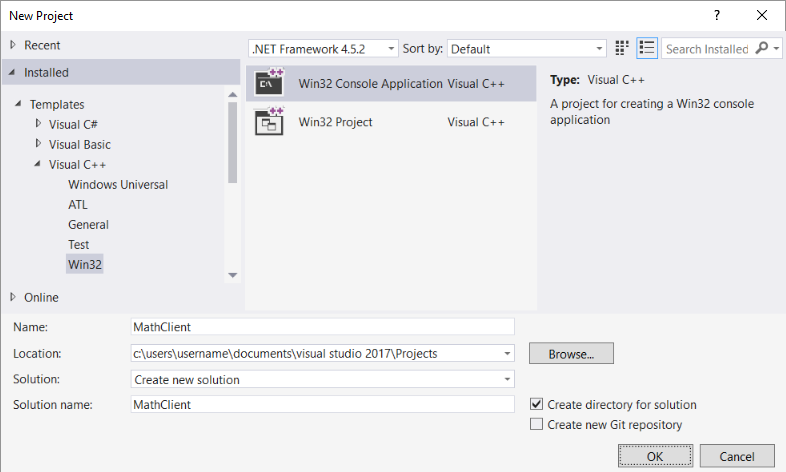3. 选择“确定” 按钮以关闭“新建项目” 对话框并启动“Win32 应用程序向导” 。 在 “Win32 应用程序向导” 对话框的 “概述” 页上，选择 “下一步” 按钮。

4. 在“应用程序设置” 页的“应用程序类型” 下，选择“控制台应用程序” （如果尚未选择）。

5. 选择 “完成” 按钮创建项目。

### 将 DLL 标头添加到包含路径

1. 右键单击“解决方案资源管理器” 中的“MathClient” 节点以打开“属性页” 对话框。

2. 在“配置”下拉框中，选择“所有配置”（如果尚未选择） 。

3. 在左窗格中，选择“配置属性”>“C/C++”>“常规” 。

4. 在属性窗格中，选择“附加包含目录” 编辑框旁的下拉控件，然后选择“编辑” 。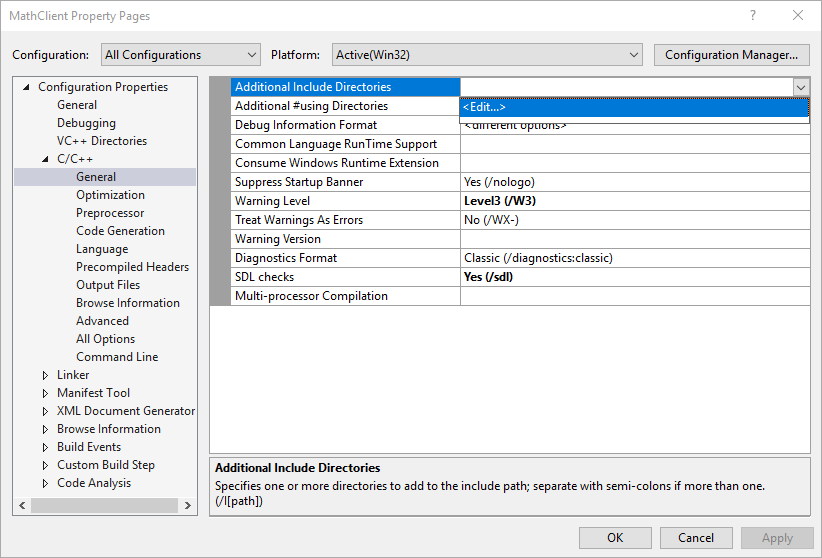5. 在“附加包含目录” 对话框的顶部窗格中双击以启用编辑控件。 或者，选择文件夹图标以创建新条目。

6. 在编辑控件中，指定指向 MathLibrary.h 头文件的位置的路径。 可选择省略号 (...) 控件浏览到正确的文件夹 。

还可将客户端源文件中的相对路径输入到包含 DLL 头文件的文件夹。 如果已按照指示将客户端项目置于 DLL 的单独解决方案中，则相对路径应如下所示：

`..\..\MathLibrary\MathLibrary`

如果 DLL 和客户端项目位于同一解决方案中，则相对路径可能如下所示：

`..\MathLibrary`

如果 DLL 和客户端项目位于其他文件夹中，请调整相对路径以进行匹配。 或者，使用省略号控件浏览文件夹。7. 在“附加包含项目”对话框中输入标头文件的路径后，选择“确定”按钮 。 在“属性页”对话框中，选择“确定”按钮以保存更改 。

``````// MathClient.cpp : Client app for MathLibrary DLL.
// #include "pch.h" Uncomment for Visual Studio 2017 and earlier
#include <iostream>
#include "MathLibrary.h"

int main()
{
// Initialize a Fibonacci relation sequence.
fibonacci_init(1, 1);
// Write out the sequence values until overflow.
do {
std::cout << fibonacci_index() << ": "
<< fibonacci_current() << std::endl;
} while (fibonacci_next());
// Report count of values written before overflow.
std::cout << fibonacci_index() + 1 <<
" Fibonacci sequence values fit in an " <<
"unsigned 64-bit integer." << std::endl;
}
``````

### 将 DLL 导入库添加到项目中

1. 右键单击“解决方案资源管理器”中的“MathClient”节点，然后选择“属性”以打开“属性页”对话框 。

2. 在“配置”下拉框中，选择“所有配置”（如果尚未选择） 。 它可确保任何属性更改同时应用于调试和发布版本。

3. 在左窗格中，选择“配置属性”>“链接器”>“输入” 。 在属性窗格中，选择“附加依赖项” 编辑框旁的下拉控件，然后选择“编辑” 。4. 在“附加依赖项”对话框中，将 MathLibrary.lib 添加到顶部编辑控件的列表中 。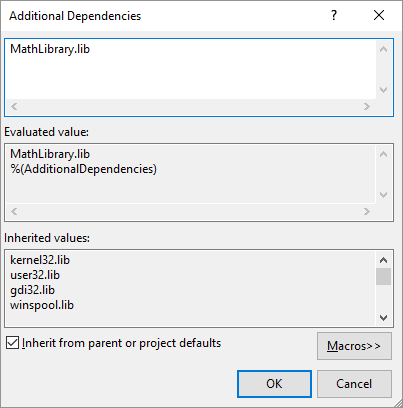5. 选择“确定” 返回到“属性页” 对话框。

6. 在左窗格中，选择“配置属性”>“链接器”>“常规” 。 在属性窗格中，选择“附加库目录” 编辑框旁的下拉控件，然后选择“编辑” 。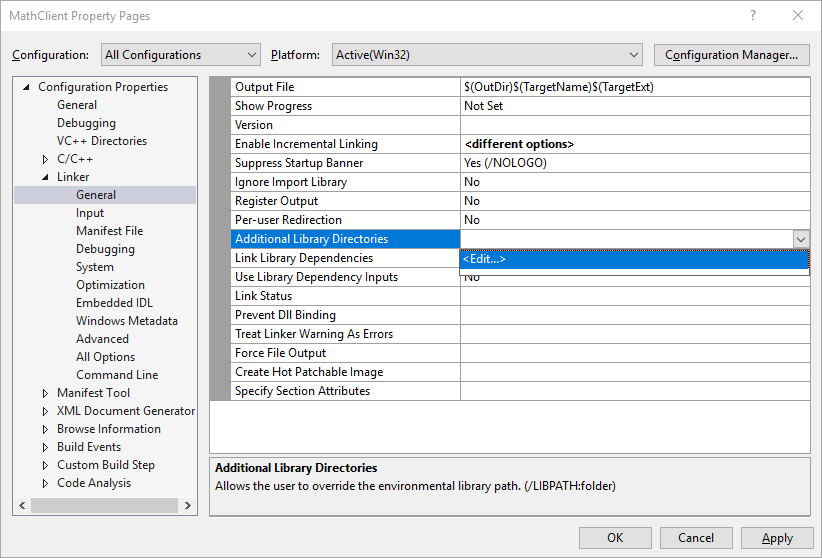7. 在“附加库目录” 对话框的顶部窗格中双击以启用编辑控件。 在编辑控件中，指定指向 MathLibrary.lib 文件位置的路径。 默认情况下，它位于 DLL 解决方案文件夹下的“Debug”文件夹中 。 如果创建发布版本，该文件会放置在“Release”文件夹中 。 可以使用 `\$(IntDir)` 宏，这样无论创建的是哪种版本，链接器都可找到 DLL。 如果已按照指示将客户端项目置于 DLL 项目的单独解决方案中，则相对路径应如下所示：

`..\..\MathLibrary\\$(IntDir)`

如果 DLL 和客户端项目位于其他位置，请调整相对路径以进行匹配。8. 在“附加库目录”对话框中输入指向库文件的路径后，选择“确定”按钮返回到“属性页”对话框 。 选择“确定”以保存属性更改 。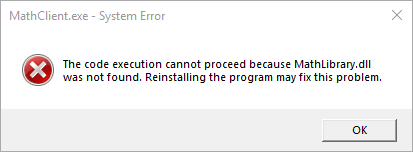### 在生成后事件中复制 DLL

1. 右键单击“解决方案资源管理器”中的“MathClient”节点，然后选择“属性”以打开“属性页”对话框 。

2. 在“配置” 下拉框中，选择“所有配置” （如果尚未选择）。

3. 在左窗格中，选择“配置属性”>“生成时间”>“后期生成事件” 。

4. 在属性窗格中，在“命令行”字段中选择编辑控件 。 如果已按照指示将客户端项目置于 DLL 项目的单独解决方案中，则输入以下命令：

`xcopy /y /d "..\..\MathLibrary\\$(IntDir)MathLibrary.dll" "\$(OutDir)"`

如果 DLL 和客户端项目在其他目录中，请更改 DLL 的相对路径以进行匹配。5. 选择“确定” 按钮以保存对项目属性所做的更改。

``````1>------ Build started: Project: MathClient, Configuration: Debug Win32 ------
1>MathClient.cpp
1>1 File(s) copied
========== Build: 1 succeeded, 0 failed, 0 up-to-date, 0 skipped ==========
``````